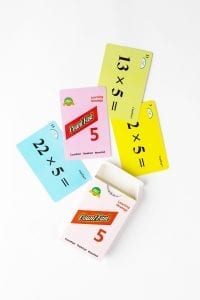# CountFast 5 Lesson Plan

####OVERVIEW & PURPOSE

Week 4 of the 3rd Grade CountFast program focuses on specific mental strategies for multiplying with the numbers 5, 15, and 50. Spend 15 minutes each day on one of the activities listed in this module.  Card decks should go home with students each day for additional practice with a parent at home. Each week, a new deck is introduced, and the previous deck is for the student to keep at home for continued practice.

#### EDUCATION STANDARDS

1   CCSS.Math.Content.3.OA.B.5
Apply properties of operations as strategies to multiply and divide.

2 NCTM Standard: develop a sense of whole numbers and represent and use them in flexible ways, including relating, composing, and decomposing numbers

#### OBJECTIVES

1. Quickly, mentally multiply by 5, 15, or 50.
2. Develop fluency with multiplication calculations.

#### MATERIALS NEEDED

1. One CountFast 5, 15, 50 card deck for each student.  This deck is for school and home use.  Discuss routine and expectations for taking home the deck and returning it to school each day.
2. One writing utensil per student, optional per teacher plans.

#### CountFast 5, 15, 50 Pack – Day 1

Teacher Model/Direct:  Use the yellow cards from the deck and teach students the fast way to multiply any number by 5.  Since 5 is half of 10, we will first multiply the number by 10 (by simply adding a zero to the end of the number), and then dividing that answer by 2 (cut in in half).  For example, 12 X 5 can be solved quickly by adding a zero to the 12 (multiply it by 10) to get 120, and then cutting 120 in half (divide by 2) to get 60.  This will take several rounds of practice with teacher modeling.

Student Activity:  Give each student a deck and ask them to take out the yellow cards.  Working with a partner, take turns holding up the cards for the partner to multiply, using the method learned in class.

Home Activity: Students will take home the deck and the “CountFast Home Connection” letter.  Students will review the yellow cards with a parent and explaining how to solve the problems quickly using the method learned in class. Parent can record how quickly the child multiplies all the cards correctly.

#### CountFast 5, 15, 50 Pack – Day 2 and 3

Teacher Model/Direct:  Using the same procedure as Day 1, review the strategy for quickly multiplying any number times 5.  Then use the blue cards to introduce how to expand on that strategy to multiply any number by 15.  Since 15 is the same as 3 X 5, first multiply the number by 3, and then use that product and follow the ‘times 5’ procedure learned on Day 1.  For example, 6 X 15 can mentally be solved by first multiplying the 6 times 3 to get 18.  Then take 18 times 10 to get 180, and cut 180 in half to get a final answer of 90.

Student Activity:  Students will work in partners to practice with the yellow and blue cards, using the mental calculation tips for multiplying by 5 and by 15.

Home Activity: Students will repeat the partner practice at home with a parent.

CountFast 5, 15, 50 Pack – Day 4 and 5

Teacher Model/Direct:  Use the pink cards from the deck.  Today, show students how to multiply any number by 50.  Since 50 is the same as 5 X 10, first multiply the number by 5.  (use the 5’s strategy learned on day 1).  Then multiply that product by 10 by simply adding a zero.  For example, to multiply 26 by 50, first multiply 26 X 5 (26 X 10 = 260.  260 divided in half is 130.)  Then take the product 130 times 10 by adding a zero.  Final answer is 1300.

Student Activity: With a partner, use the pink cards to practice multiplying by 50, using the strategy learned in the lesson.

Home Activity: Students will review the activities for multiplying by 50, using the strategy learned in class.

#### Instructional video

Stay SharpFree flash card decks to keep kids entertained and sharp!

To help you keep your kids math sharp during this crisis, we're giving you 3 free CountFast flash card decks for just \$6 in shipping.  Just have them take the quick quiz for their grade at the link below and you'll get a discount code to use on any three decks.